# Fomo3D: Ponzi or Gambling?

#### I. Background of Blockchain-based Gambling

Gambling is never a new idea. However, there are many limits of traditional gambling. For example, the games are controlled by the operator, who will have strong incentive to roll away all the invested capital. Besides, gambling has always been strictly controlled and monitored in most countries, and therefore limited access has been given to the general public.

Known mostly for its decentralization feature, blockchain has found itself a good application in gambling. Many companies have been building gambling or prediction platforms using the blockchain technology. These blockchain-based gambling platforms are usually built on Ethereum with its smart contract. Once the code has been written and implemented on smart contract by the game’s developers and the game has started, the game rules will never be changed unless the developers write another smart contract (start a new game) on Ethereum. Once the game started, the smart contract on Ethereum will work like a “gambling machine” or “unmanned casino” where selling tickets and paying rewards are completed by the smart contract itself according to the preset rules automatically. Therefore, these platforms have attracted many players since the decentralization feature has implied a higher credibility or level of fairness.

#### II. Rules of the Fomo3D GameFomo3D is a lottery game in which the grand prize winner is the last person to purchase a key – a lottery ticket – using ETH (a crypto) before the countdown timer runs down to zero. During a round, a timer is constantly counting down to zero. Every time a key is purchased, the buyer becomes the new “holder of the private keys” and additional time is added to the countdown. The price of the keys will get slightly more expensive with each purchase. When the timer reaches zero, the last person to have bought a key wins the round. At the end of the round, the ETH in the pot (the reward pool) is divvied up.

The basic rules include:

1. Each key purchased adds 30 seconds to the round timer, to a hard cap of 24 hours;
2. Time is added per-key, rounded down. So 2.5 keys = 60 seconds;
3. Key price scaling is very slow and gradual, increasing slightly on each purchase (see detailed price function in the next section);
4. The pot (reward pool) will be divided according to the following schedule after the final key purchaser is determined :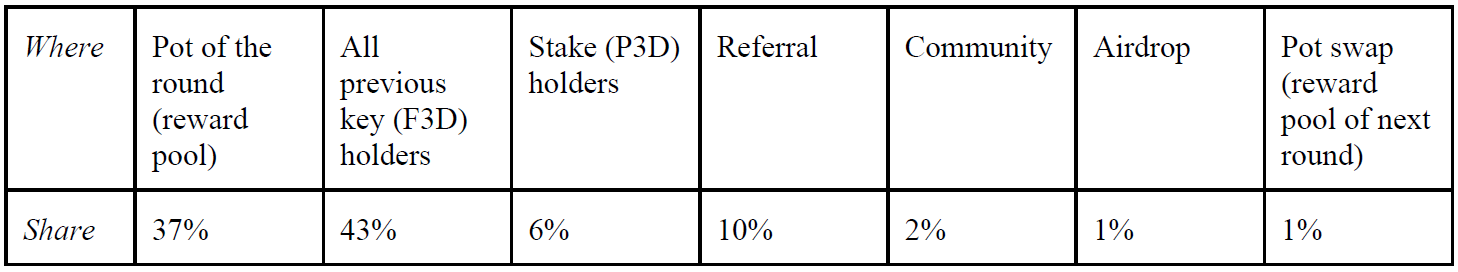1. The pot (reward pool) will be divided after the final key purchaser is determined according to the following schedule: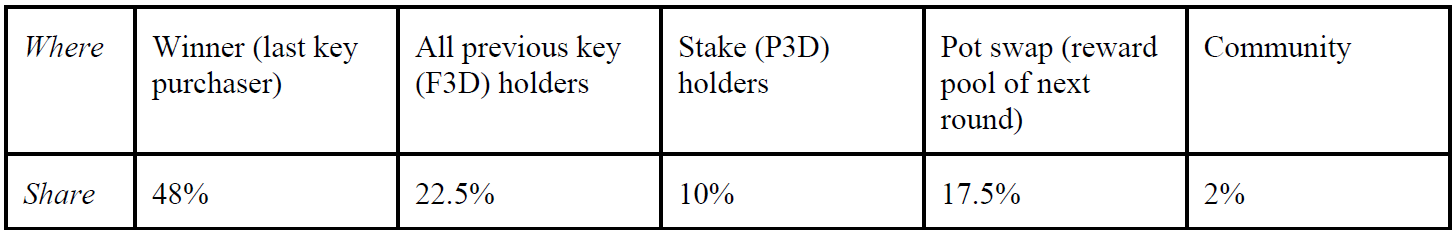#### III. Price Function

By examining the code of Fomo3D project, which is open-sourced, we find the price function code:

``` * @dev calculates how much eth would be in contract given a number of keys

* @param _keys number of keys "in contract"

* @return eth that would exists

function eth(uint256 _keys)

{

return ((78125000).mul(_keys.sq()).add(((149999843750000).mul(_keys.mul(1000000000000000000))) / (2))) / ((1000000000000000000).sq());

}```

In short, there is a function between the total invested ETH and the total number of keys issued. According to the code, the function between the total invested ETH (Q) and the total number of keys (K) is:

Total invested ETH (Q)  = (78125000 * K^2 + 74999921875000 * K * 10^18) / 10^36.

We can simplify the equation as follows: Q (K) = a * K^2 + b * K, where a = 78125000/10^36, b = 74999921875000/10^18

The price of key number n will be:

f(n) = Q(n) – Q(n-1) = (a * n^2 + b * n) – (a * (n – 1)^2 + b * (n – 1)) = b – a + 2a * n

Therefore, the price function will be:

f(n) = b – a + 2a * n

where a = 78125000/10^36, b = 74999921875000/10^18.

#### IV. Strategy Analysis

Since whether a player wins or not will depend on the action of the next player, if any, we would like to examine the potential strategies of any player. Assume there is a player (n) and player (n +1), and we get the payoff matrix as follows: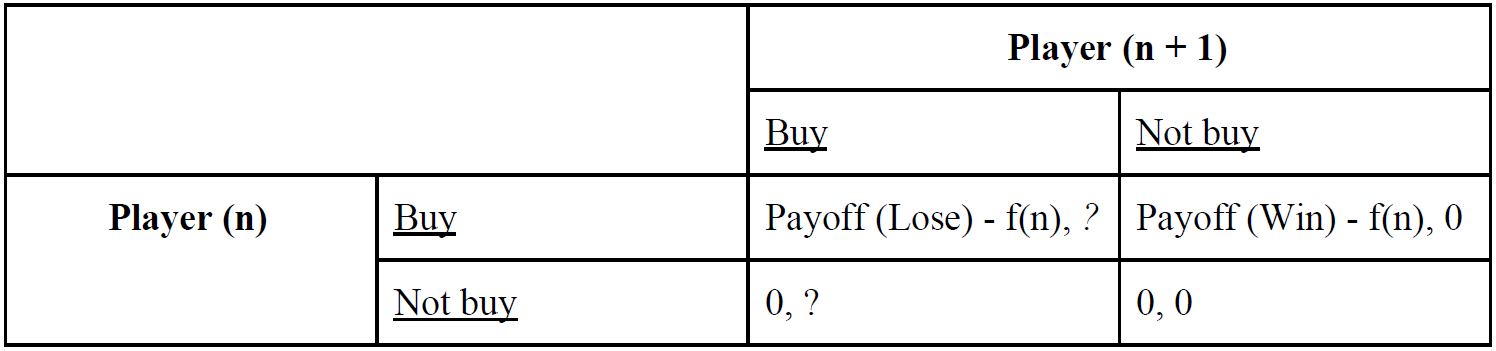To understand what strategies a player can choose, we first denote the variables as follows: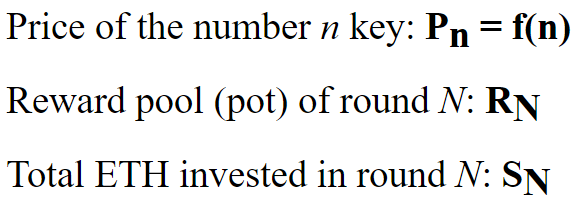According to the rules mentioned, we can get the following equations for R: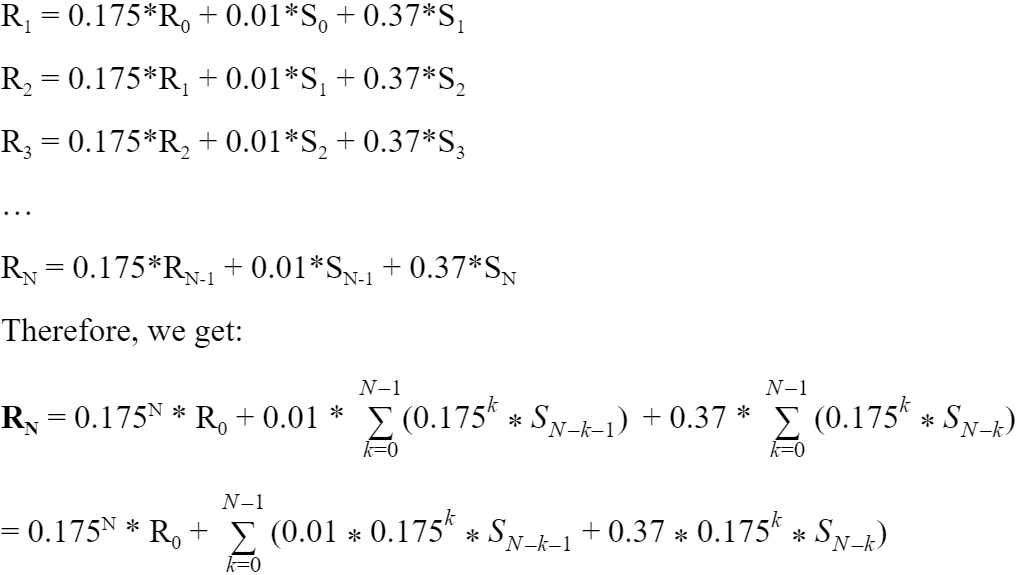According to our discussions above, we get the price function f(n) as follows:

f(n) = b – a + 2a * n

where a = 78125000/10^36, b = 74999921875000/10^18.

Now we examine round N and evaluate the payoffs in “win” and “lost” cases: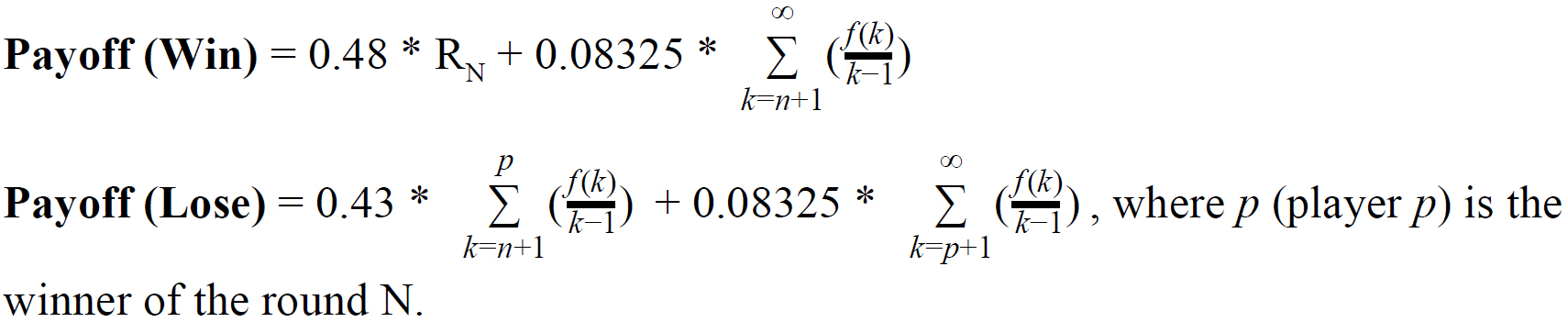Assume the player n has x probability to win and (1 – x) probability to lose, which means the next player (n + 1 player) has x probability to not buy and (1 – x) probability to buy.

Therefore, the expected total payoff for player n in round N should be: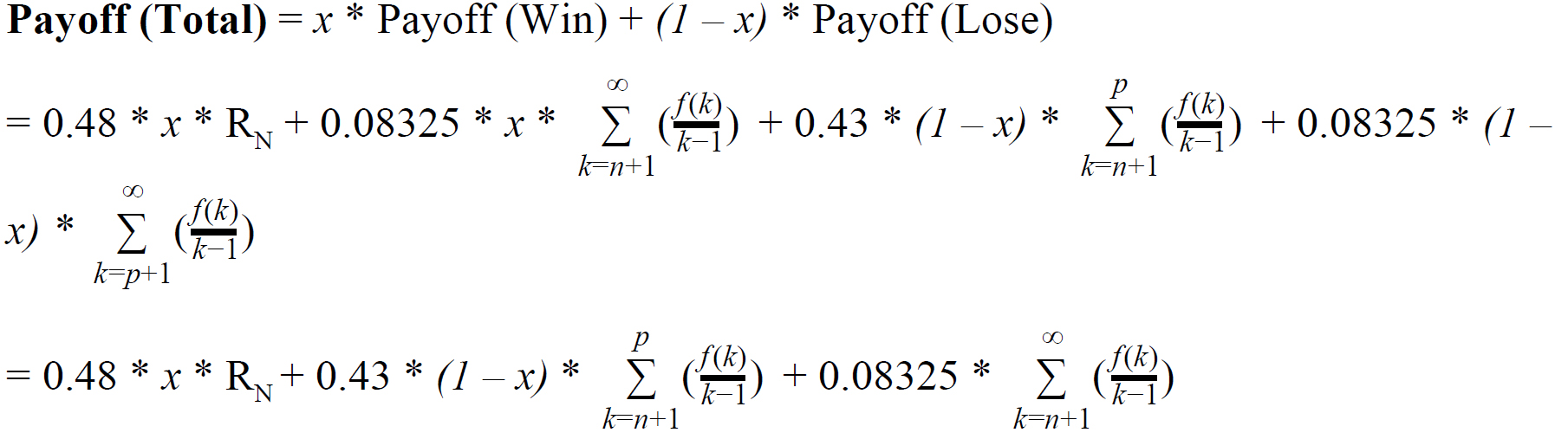where p (player p) is the winner of the round N if player n is not the winner.

Clearly, if f(n) <= Payoff (Total), then a player has incentives to participate.

Scenario 1: when n and N are very small

When both n and N are very small, RN is very small comparing to the other parts of the payoff, if we assume the games can happen many rounds after round N. Instead, the payoff from the revenue share from the following rounds, which is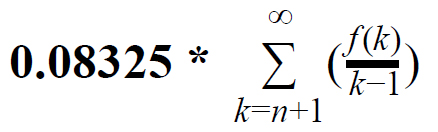, will be much larger than the payoff from winning the current round (the first two parts).

Therefore, it is obvious that f(n) <=will hold when both n and N are very small. Hence a player has a strong incentive to purchase the keys in the early rounds of the game, which is more like a Ponzi scheme.

Scenario 2: when n and N are very large

In this situation, we will wonder if there will be an end of the game where no rational investor will participate in the next round since the price of keys becomes higher than the potential payoff.

Case 1: If there is not such end, the game will continue forever since the potential total payoff will always be higher than the price of key.

Case 2: If there is such an end, we assume round N is the last round of the game. Player n should have a non-negative expected total payoff after deducting his/her cost of purchasing a key, while Player n + 1 should expect a negative total payoff after deducting the participation cost.

Now we try to evaluate if case 2 will exist.

According to the discussions above, the total payoff before deducting the cost will be: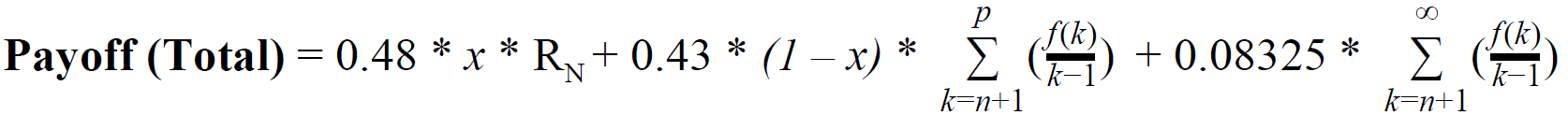Since both n and N are very huge and this is the last round, we can simplify the equation as follows:

####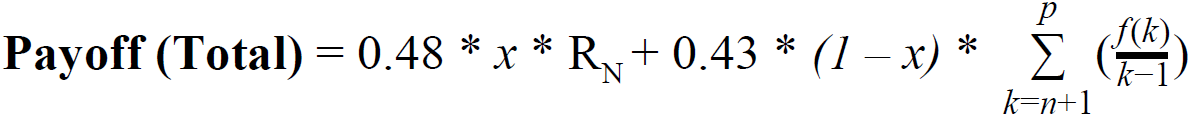Since player n knows that player n + 1 will not participate in this game for sure due to the negative after-cost payoff, x equals to 1 here. Therefore, the total after-cost payoff is: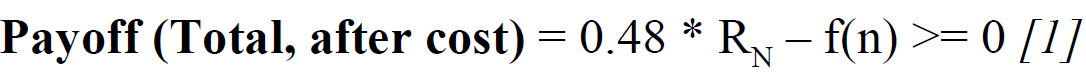In another way, we need to prove that: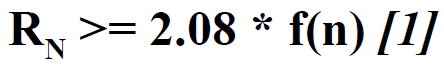If we assume there are k players participating in round N, according to the price function f(n), we can get that: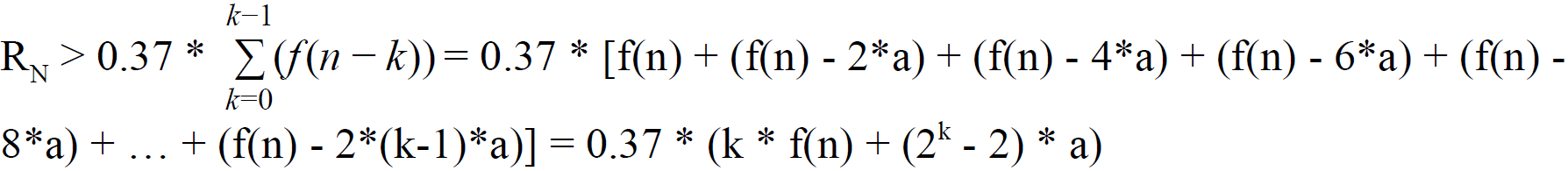We need to verify if 0.37 * (k * f(n) + (2^k – 2) * a) > 2.08 * f(n)

Therefore, we need to prove that:

(2^k – 2) * a > (5.62 – k) * f(n) 

Apparently, when k > 5, the inequation  will hold since both a and f(n) will always be positive. (Some more efforts will be needed to prove that when k <=5, the inequation  still holds)

Therefore, inequation  holds.

At the same time, we need to ensure that the total after-cost payoff for player n + 1 is negative: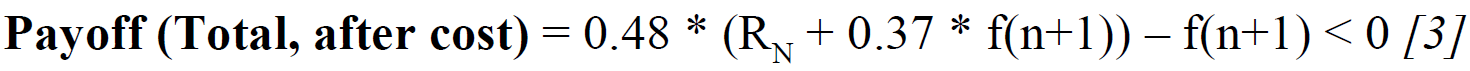We simplify the inequation  as: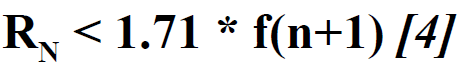Similarly, if we assume there are k players participating in round N, according to the price function f(n), we can get that: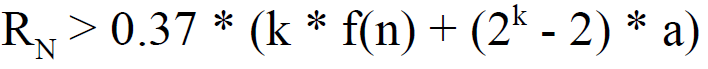We need to prove that at least: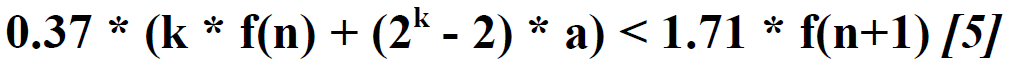which means: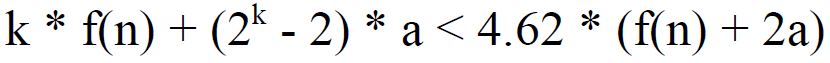In short, we need to prove: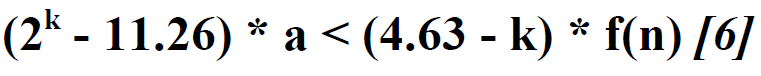Apparently, when k  >= 5, inequation  will not hold. Therefore, inequation  will almost never hold (some more efforts are needed to prove that the corner cases will not hold when k < 5).

Therefore, the end of the game will never come, indicating that a rational player will always participate in this game once it is started.

Therefore, if all players expect new players to join afterwards (x = 0), the actual payoff will be: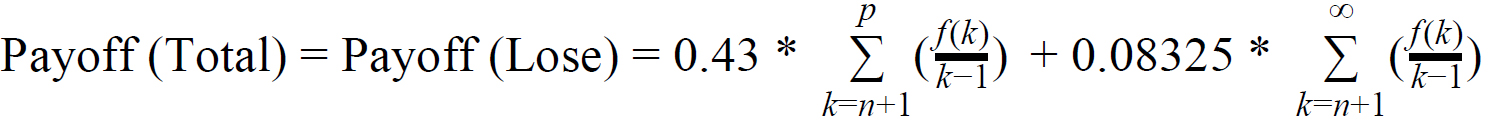If one round never ends, the total expected after-cost payoff for each player is: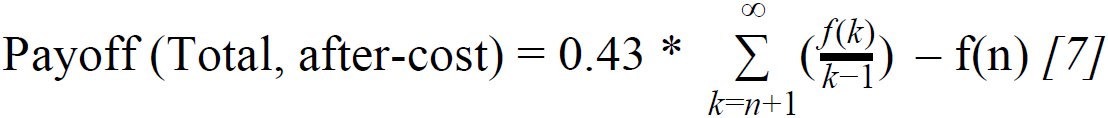If one round accidentally ends, since n has already been be very large, the first part of the payoff will be very small. Therefore, the total expected after-cost payoff for a player is: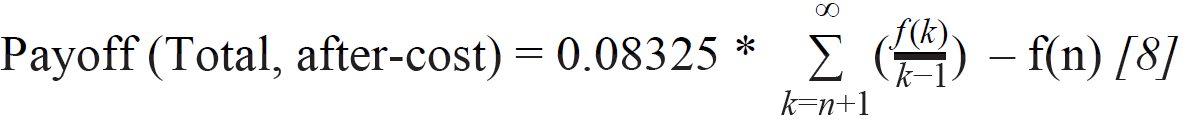Therefore, in the “late” stage, it is still a Ponzi scheme, since there will never be a “late” stage in this infinite game and the actual payoff will mostly come from the key sales from the following players. (Though it is plausible that  and  will always be positive since the first part will be positive infinity, some more efforts will be needed to prove it.)

#### V. Conclusion

Therefore, unlike what many people believe, the game does not become a gambling game even in the “late” stage since it is highly likely that the game will never come to an end. The game is an endless Ponzi scheme even after the game has started many rounds. However, the payoff may not be as high as that for the earlier key holders.

However, these conclusions are made based on a big assumption that all investors/players are rational. In real life, there can be a big group of irrational investors who will not play even knowing there is a positive expected payoff or who play even knowing there is a negative expected payoff. These irrational investors/players will make the game more complicated.

 Blockgeeks, “Smart Contracts: The Blockchain Technology That Will Replace Lawyers”: https://blockgeeks.com/guides/smart-contracts/

 Wikipedia, “Ethereum”: https://en.wikipedia.org/wiki/Ethereum

 There are four splitting schedule options called “Snek”, “Whale”, “Bull”, and “Bear”. We assume that equal portion of participants will choose each of these schedules to simplify our analysis. Therefore, we aggregated the four schedules and put the average share distribution number in this analysis. See details:

https://fomo3d.hostedwiki.co/pages/Fomo3D%20Explained#the-teams

 Ibid

This site uses Akismet to reduce spam. Learn how your comment data is processed.

This post doesn't have any comment. Be the first one!